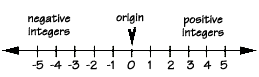We can use the number line as a model to help us visualize adding and subtracting of signed integers. Just think of addition and subtraction as directions on the number line. There are also several rules and properties that define how to perform these basic operations.

To add integers having the same sign, keep the same sign and add the absolute value of each number.

To add integers with different signs, keep the sign of the number with the largest absolute value and subtract the smallest absolute value from the largest.

Subtract an integer by adding its opposite.

Watch out! The negative of a negative is the opposite positive number. That is, for real numbers,

-(-a) = +aHere's how to add two positive integers:

4 + 7 = ?

If you start at positive four on the number line and move seven units to the right, you end up at positive eleven. Also, these integers have the same sign, so you can just keep the sign and add their absolute values, to get the same answer, positive eleven.

Here's how to add two negative integers:

-4 + (-8) = ?

If you start at negative four on the number line and move eight units to the left, you end up at negative twelve. Also, these integers have the same sign, so you can just keep the negative sign and add their absolute values, to get the same answer, negative twelve.

Here's how to add a positive integer to a negative integer:

-3 + 6 = ?

If you start at negative three on the real number line and move six units to the right, you end up at positive three. Also, these integers have different signs,

so keep the sign from the integer having the greatest absolute value and subtract the smallest absolute value from the largest.

Subtract three from six and keep the positive sign, again giving positive three.

Here's how to add a negative integer to a positive integer:

5 + (-8) = ?

If you start at positive five on the real number line and move eight units to the left, you end up at negative three. Also, these integers have different signs, so keep the sign from the integer having the greatest absolute value and subtract the smallest absolute value from the largest, or subtract five from eight and keep the negative sign, again giving negative three.

To subtract a number, add its opposite:

5 - 8 = ?

Because they give the same result, you can see that subtracting eight from five is equivalent to adding negative eight to positive five. The answer is - 3.

To subtract a number, add its opposite:

-3 - (-6) = ?

Because they give the same result, you can see that subtracting negative six from negative three is equivalent to adding positive six to negative three. The answer is 3.Homework Help | Pre-Algebra | NumbersEmail this page to a friendSearch·  Place value·  Decimal numbers·  Estimating and     rounding·  Adding / subtracting     decimals·  Multiplying decimals·  Dividing decimals·  Percent·  Exponents·  Square roots·  Signed integers·  Adding and     subtracting integers·  Multiplying and     dividing integers·  Properties of integersFirst Glance In Depth Examples WorkoutAdding and Subtracting Integers